Author: Oscar Cronquist Article last updated on November 06, 2018

Question: How do I sort a range alphabetically using excel array formula?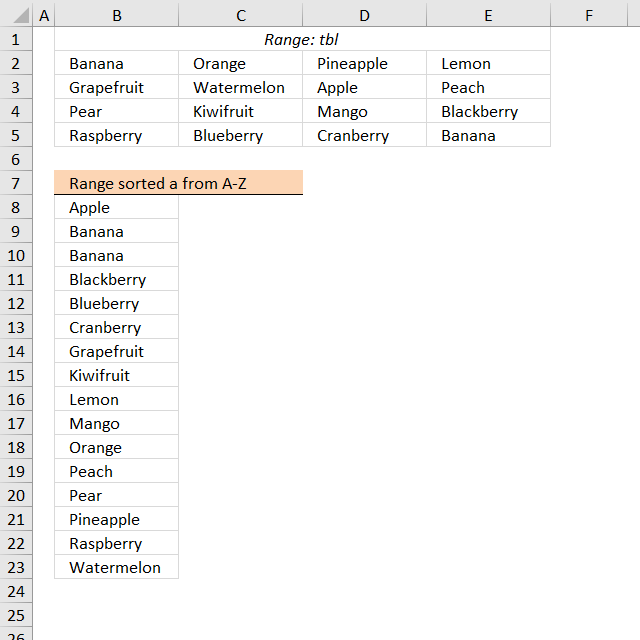Cell range \$B\$2:\$E\$5 contains text values in random order, the formula in cell B8 extracts values sorted from A to Z.

Array formula in B8:

=INDEX(\$B\$2:\$E\$5, MIN(IF(SMALL(COUNTIF(\$B\$2:\$E\$5, "<"&\$B\$2:\$E\$5), ROWS(\$B\$8:B8))=COUNTIF(\$B\$2:\$E\$5, "<"&\$B\$2:\$E\$5), ROW(\$B\$2:\$E\$5)-MIN(ROW(\$B\$2:\$E\$5))+1)), MATCH(SMALL(COUNTIF(\$B\$2:\$E\$5, "<"&\$B\$2:\$E\$5), ROWS(\$B\$8:B8)), COUNTIF(\$B\$2:\$E\$5, "<"&INDEX(\$B\$2:\$E\$5, MIN(IF(SMALL(COUNTIF(\$B\$2:\$E\$5, "<"&\$B\$2:\$E\$5), ROWS(\$B\$8:B8))=COUNTIF(\$B\$2:\$E\$5, "<"&\$B\$2:\$E\$5), ROW(\$B\$2:\$E\$5)-MIN(ROW(\$B\$2:\$E\$5))+1)), , 1)), 0))

Filter unique distinct values sorted from A to Z with no blanks from a multi-column and multi-row cell range:

Extract a unique distinct list sorted alphabetically and ignore blanks from a range

This is an answer to a question in this blog post: Extract a unique distinct list sorted from A-Z from […]

#### How to enter an array formula

1. Double click on cell B8
2. Copy an paste above formula
3. Press and hold CTRL + SHIFT simultaneously
4. Press Enter once

Learn how to filter unique distinct values from a multi-column and multi-row cell range:

Extract unique distinct values from a multi-column cell range

Question: I have cell values spanning over several columns and I want to create a unique list from that range. […]

#### How to implement array formula to your workbook

If your list starts at, for example, F2. Change \$B\$8:B8  in the above formula to F2:\$F\$2.

The following article demonstrates how to filter a unique distinct list sorted from A to Z, from a multi-column and multi-row cell range:

Extract a unique distinct list sorted from A-Z from range

The image above shows an array formula in cell B8 that extracts unique distinct values sorted alphabetically from cell range […]

#### How to copy array formula

Copy cell B8 and paste to cells below.

How to filter duplicate values from a multi-column and multi-row cell range:

Extract duplicates from a range

The following array formula in cell B11 extracts duplicates from cell range B3:E8, only one instance of each duplicate is […]

### Explaining formula in cell B8

#### Step 1 - Rank values based on their relative position if they were sorted

The COUNTIF function counts cells based on a condition, however, in this case, I am using a cell range instead of a single cell in the second argument.

Also, the ampersand & concatenates each value with a less than sign. This makes the COUNTIF function compare each value against the others, the number returned represents the corresponding values position if the list were sorted.

COUNTIF(\$B\$2:\$E\$5,"<"&\$B\$2:\$E\$5)

returns {1, 10, 13, 8; 6, 15, 0, 11; 12, 7, 9, 3; 14, 4, 5, 1}.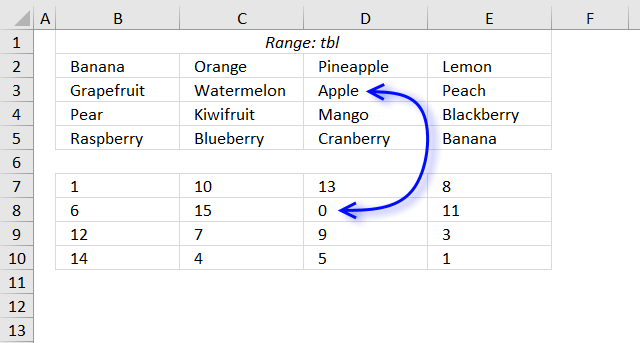The image above shows the array in cell range B7:E10, "Apple" is 0 (zero) which is the first position if the list were sorted.

#### Step 2 - Extract the k-th smallest value in array

The SMALL function lets you get the k-th smallest value from a cell range or array.

SMALL(array, k)

SMALL(COUNTIF(\$B\$2:\$E\$5, "<"&\$B\$2:\$E\$5), ROWS(\$B\$8:B8))

The ROWS function allows you to keep track of the number of cells, the cell reference is expanding as we copy the formula and paste to cells below.

SMALL({1, 10, 13, 8; 6, 15, 0, 11; 12, 7, 9, 3; 14, 4, 5, 1}, ROWS(\$B\$8:B8))

becomes

SMALL({1, 10, 13, 8; 6, 15, 0, 11; 12, 7, 9, 3; 14, 4, 5, 1}, 1) and returns 0 (zero).

#### Step 3 - Compare value to array

The logical expression in the following IF function determines if the value of the position corresponding to the k-th smallest value will be a row number or FALSE.

IF(SMALL(COUNTIF(\$B\$2:\$E\$5, "<"&\$B\$2:\$E\$5), ROWS(\$B\$8:B8))=COUNTIF(\$B\$2:\$E\$5, "<"&\$B\$2:\$E\$5), ROW(\$B\$2:\$E\$5)-MIN(ROW(\$B\$2:\$E\$5))+1)

becomes

IF(0=COUNTIF(\$B\$2:\$E\$5, "<"&\$B\$2:\$E\$5), ROW(\$B\$2:\$E\$5)-MIN(ROW(\$B\$2:\$E\$5))+1)

becomes

IF(0={1, 10, 13, 8; 6, 15, 0, 11; 12, 7, 9, 3; 14, 4, 5, 1}, ROW(\$B\$2:\$E\$5)-MIN(ROW(\$B\$2:\$E\$5))+1)

becomes

IF({FALSE, FALSE, FALSE, FALSE;FALSE, FALSE, TRUE, FALSE;FALSE, FALSE, FALSE, FALSE;FALSE, FALSE, FALSE, FALSE}, ROW(\$B\$2:\$E\$5)-MIN(ROW(\$B\$2:\$E\$5))+1)

becomes

IF({FALSE, FALSE, FALSE, FALSE;FALSE, FALSE, TRUE, FALSE;FALSE, FALSE, FALSE, FALSE;FALSE, FALSE, FALSE, FALSE}, {1;2;3;4})

and returns {FALSE, FALSE, FALSE, FALSE;FALSE, FALSE, 2, FALSE;FALSE, FALSE, FALSE, FALSE;FALSE, FALSE, FALSE, FALSE}.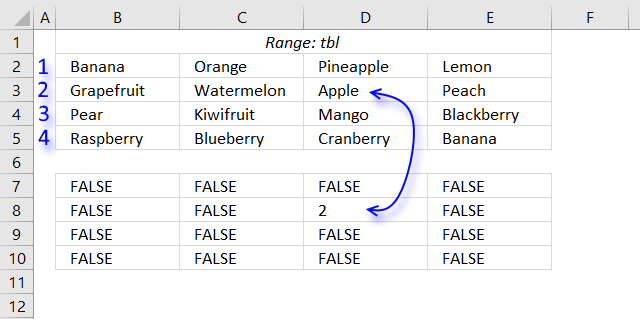#### Step 4 - Extract the smallest number from array

The MIN function ignores boolean values TRUE and FALSE.

MIN(IF(SMALL(COUNTIF(\$B\$2:\$E\$5, "<"&\$B\$2:\$E\$5), ROWS(\$B\$8:B8))=COUNTIF(\$B\$2:\$E\$5, "<"&\$B\$2:\$E\$5), ROW(\$B\$2:\$E\$5)-MIN(ROW(\$B\$2:\$E\$5))+1))

becomes

MIN({FALSE, FALSE, FALSE, FALSE;FALSE, FALSE, 2, FALSE;FALSE, FALSE, FALSE, FALSE;FALSE, FALSE, FALSE, FALSE})

and returns 2. We now know that the first value to be extracted is located on row 2 relative to cell range \$B\$2:\$E\$5.

#### Step 5 - Find column

In this case, the MATCH function returns the relative position horizontally of the value we are looking for.

MATCH(SMALL(COUNTIF(\$B\$2:\$E\$5,"<"&\$B\$2:\$E\$5),ROWS(\$B\$8:B8)),COUNTIF(\$B\$2:\$E\$5,"<"&INDEX(\$B\$2:\$E\$5,MIN(IF(SMALL(COUNTIF(\$B\$2:\$E\$5,"<"&\$B\$2:\$E\$5),ROWS(\$B\$8:B8))=COUNTIF(\$B\$2:\$E\$5,"<"&\$B\$2:\$E\$5),ROW(\$B\$2:\$E\$5)-MIN(ROW(\$B\$2:\$E\$5))+1)),,1)),0)

becomes

MATCH(SMALL({1, 10, 13, 8; 6, 15, 0, 11; 12, 7, 9, 3; 14, 4, 5, 1},ROWS(\$B\$8:B8)),COUNTIF(\$B\$2:\$E\$5,"<"&INDEX(\$B\$2:\$E\$5,MIN(IF(SMALL(COUNTIF(\$B\$2:\$E\$5,"<"&\$B\$2:\$E\$5),ROWS(\$B\$8:B8))=COUNTIF(\$B\$2:\$E\$5,"<"&\$B\$2:\$E\$5),ROW(\$B\$2:\$E\$5)-MIN(ROW(\$B\$2:\$E\$5))+1)),,1)),0)

becomes

MATCH(SMALL({1, 10, 13, 8; 6, 15, 0, 11; 12, 7, 9, 3; 14, 4, 5, 1},1),COUNTIF(\$B\$2:\$E\$5,"<"&INDEX(\$B\$2:\$E\$5,MIN(IF(SMALL(COUNTIF(\$B\$2:\$E\$5,"<"&\$B\$2:\$E\$5),ROWS(\$B\$8:B8))=COUNTIF(\$B\$2:\$E\$5,"<"&\$B\$2:\$E\$5),ROW(\$B\$2:\$E\$5)-MIN(ROW(\$B\$2:\$E\$5))+1)),,1)),0)

becomes

MATCH(0,COUNTIF(\$B\$2:\$E\$5,"<"&INDEX(\$B\$2:\$E\$5,MIN(IF(SMALL(COUNTIF(\$B\$2:\$E\$5,"<"&\$B\$2:\$E\$5),ROWS(\$B\$8:B8))=COUNTIF(\$B\$2:\$E\$5,"<"&\$B\$2:\$E\$5),ROW(\$B\$2:\$E\$5)-MIN(ROW(\$B\$2:\$E\$5))+1)),,1)),0)

I will be using the INDEX function to extract the values we need based on a row number.

MATCH(0,COUNTIF(\$B\$2:\$E\$5,"<"&INDEX(\$B\$2:\$E\$5,MIN(IF(SMALL(COUNTIF(\$B\$2:\$E\$5,"<"&\$B\$2:\$E\$5),ROWS(\$B\$8:B8))=COUNTIF(\$B\$2:\$E\$5,"<"&\$B\$2:\$E\$5),ROW(\$B\$2:\$E\$5)-MIN(ROW(\$B\$2:\$E\$5))+1)),,1)),0)

becomes

MATCH(0,COUNTIF(\$B\$2:\$E\$5,"<"&INDEX(\$B\$2:\$E\$5,2,,1)),0)

becomes

MATCH(0,COUNTIF(\$B\$2:\$E\$5,"<"&B3:E3),0)

becomes

MATCH(0,{6,15,0,11},0)

and returns 3. The value we are looking for is in column 3 relative to cell range \$B\$2:\$E\$5.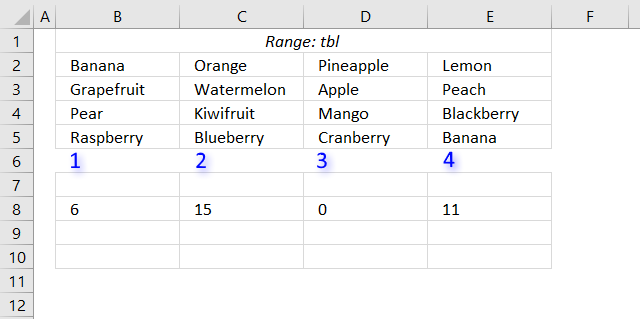#### Step 6 - Return value based on row and column

INDEX(\$B\$2:\$E\$5, MIN(IF(SMALL(COUNTIF(\$B\$2:\$E\$5, "<"&\$B\$2:\$E\$5), ROWS(\$B\$8:B8))=COUNTIF(\$B\$2:\$E\$5, "<"&\$B\$2:\$E\$5), ROW(\$B\$2:\$E\$5)-MIN(ROW(\$B\$2:\$E\$5))+1)), MATCH(SMALL(COUNTIF(\$B\$2:\$E\$5, "<"&\$B\$2:\$E\$5), ROWS(\$B\$8:B8)), COUNTIF(\$B\$2:\$E\$5, "<"&INDEX(\$B\$2:\$E\$5, MIN(IF(SMALL(COUNTIF(\$B\$2:\$E\$5, "<"&\$B\$2:\$E\$5), ROWS(\$B\$8:B8))=COUNTIF(\$B\$2:\$E\$5, "<"&\$B\$2:\$E\$5), ROW(\$B\$2:\$E\$5)-MIN(ROW(\$B\$2:\$E\$5))+1)), , 1)), 0))

becomes

INDEX(\$B\$2:\$E\$5, 2, 3)

and returns "Apple" in cell B8.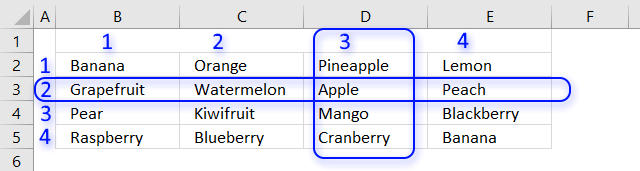Range sorted from A to Z.xlsx

This post shows you how to filter a multi-column and multi-row cell range based on frequency:

Sort a range based on value frequency

The formula in cell B8 extracts a list sorted based on frequency. Array formula in B8: =TEXTJOIN("", TRUE, IF(MIN(IF((MAX(IF(COUNTIF(\$B\$7:B7, \$B\$2:\$E\$5)=0, […]

Recommended article

How to enter an array formula

Array formulas allows you to do advanced calculations not possible with regular formulas.

Recommended article

Sort a table with an array formula

The sorted table to the right is created with an array formula using the data in the table to the left. Array […]

This blog article is one out of five articles on the same subject.

Sort text cells alphabetically from two columns

Table of Contents Sort text from two columns combined (array formula) Sort text from multiple cell ranges combined (user defined […]

Create a drop down list containing only unique distinct alphabetically sorted text values

Question: How do I create a drop-down list with unique distinct alphabetically sorted values? Table of contents Sort values using […]